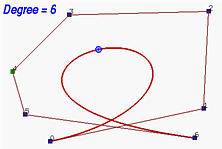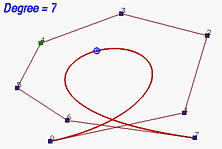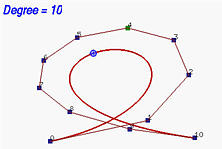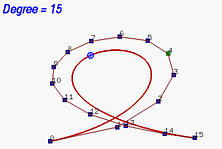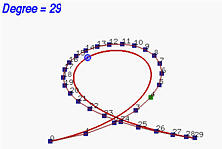# Degree Elevation of a Bézier CurveMany applications that involve two or more Bézier curves require all involved curves to have the same degree. Moreover, although higher degree Bézier curves require longer time to process, they do have higher flexibility for designing shapes. Therefore, it would be very helpful to increase the degree of a Bézier curve without changing its shape. Note that "without changing the curve's shape" is the key point; otherwise, just increasing the degree of a Bézier curve does not make any practical sense. Increasing the degree of a Bézier curve without changing its shape is referred to as degree elevation. In what follows, only an algorithm will be discussed.

Suppose we have a Bézier curve of degree n defined by n + 1 control points P0, P1, P2, ..., Pn and we want to increase the degree of this curve to n + 1 without changing its shape. Since a degree n + 1 Bézier curve is defined by n + 2 control points, we need to find such a new set of control points. Obviously, P0 and Pn must be in the new set because the new curve also passes through them. Therefore, what we need is only n new control points. Let the new set of control points be Q0, Q1, Q2, ..., Qn+1. As mentioned above, Q0 = P0 and Qn+1 = Pn. The other control points are computed as follows: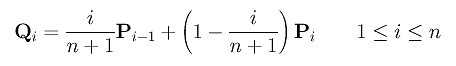If you are not comfortable with the above general formula, the following are formulae for each control points from Q1 to Qn.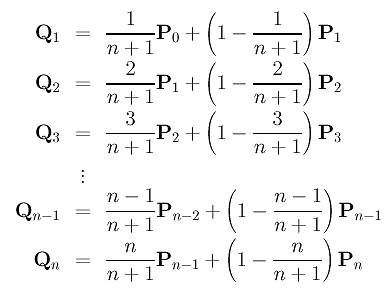Each leg of the original polyline contains exactly one new control point. More precisely, leg Pi-1Pi contains new control point Qi. Recall from the discussion of de Casteljau's algorithm that a point C on a line segment AB that divides AB in a ratio of u:1-u can be written as C = (1 - u)A + uB. From the formulae for the new control points, we see that Qi divides the segment Pi-1Pi in a ratio of 1 - i/(n+1):i/(n+1). However, unlike de Casteljau's algorithm, this ratio is not a constant but varying with the value of i. This computation is very similar to that of de Casteljau's algorithm, though.

Once the new set of control points is obtained, the original set can be discarded. Since each leg of the original control polyline contains a new control point, the process of replacing the old set with the new one can be viewed as cutting off the corners at the original control points. The following figure illustrates this corner-cutting effect. The figure shows a Bézier curve of degree 4 whose control points are shown in red rectangles and control polyline in blue dashed line segments. After increasing its degree to 5, the new control polyline is shown in solid line segments. It is clear that all corners are cut. The left table gives the ratio on each leg of the original control polyline.

 i 1 - i/(n+1) 1 0.8 2 0.6 3 0.4 4 0.2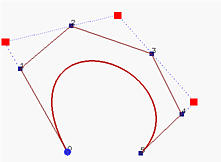Note that degree elevation can be used repeatedly as long as your system permits. Note also that as the degree increases the number of control points increases. Moreover, the new control polyline moves toward the curve. In the following figures, we start with a Bézier curve of degree 6 with 7 control points. Then, its degree is increased to 7, 8, 10, 15 and 29. As you can see from the figures, the shape of the curve is not changed as its degree increases and the control polyline moves closer and closer to the curve. Eventually, as the degree keeps increasing to infinity, the control polyline approaches to the curve and has it as a limiting position.